Open in App
Not now

# How to normalize an array in NumPy in Python?

• Difficulty Level : Easy
• Last Updated : 21 Nov, 2022

In this article, we are going to discuss how to normalize 1D and 2D arrays in Python using NumPy. Normalization refers to scaling values of an array to the desired range.

## Normalization of 1D-Array

Suppose, we have an array = [1,2,3] and to normalize it in range [0,1] means that it will convert array [1,2,3] to [0, 0.5, 1] as 1, 2 and 3 are equidistant.

`Array [1,2,4] -> [0, 0.3, 1]`

This can also be done in a Range i.e. instead of [0,1], we will use [3,7].

Now,

`Array [1,2,3] -> [3,5,7]`

and

`Array [1,2,4] -> [3,4.3,7]`

Let’s see examples with code

Example 1:

## Python3

 `# import module``import` `numpy as np` `# explicit function to normalize array``def` `normalize(arr, t_min, t_max):``    ``norm_arr ``=` `[]``    ``diff ``=` `t_max ``-` `t_min``    ``diff_arr ``=` `max``(arr) ``-` `min``(arr)   ``    ``for` `i ``in` `arr:``        ``temp ``=` `(((i ``-` `min``(arr))``*``diff)``/``diff_arr) ``+` `t_min``        ``norm_arr.append(temp)``    ``return` `norm_arr` `# gives range starting from 1 and ending at 3 ``array_1d ``=` `np.arange(``1``,``4``)``range_to_normalize ``=` `(``0``,``1``)``normalized_array_1d ``=` `normalize(array_1d,``                                ``range_to_normalize[``0``],``                                ``range_to_normalize[``1``])` `# display original and normalized array``print``(``"Original Array = "``,array_1d)``print``(``"Normalized Array = "``,normalized_array_1d)`

Output: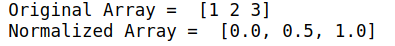Example 2:

Now, Lets input array is [1,2,4,8,10,15] and range is again [0,1]

## Python3

 `# import module``import` `numpy as np` `# explicit function to normalize array``def` `normalize(arr, t_min, t_max):``    ``norm_arr ``=` `[]``    ``diff ``=` `t_max ``-` `t_min``    ``diff_arr ``=` `max``(arr) ``-` `min``(arr)``    ``for` `i ``in` `arr:``        ``temp ``=` `(((i ``-` `min``(arr))``*``diff)``/``diff_arr) ``+` `t_min``        ``norm_arr.append(temp)``    ``return` `norm_arr` `# assign array and range``array_1d ``=` `[``1``, ``2``, ``4``, ``8``, ``10``, ``15``]``range_to_normalize ``=` `(``0``, ``1``)``normalized_array_1d ``=` `normalize(``    ``array_1d, range_to_normalize[``0``],``  ``range_to_normalize[``1``])` `# display original and normalized array``print``(``"Original Array = "``, array_1d)``print``(``"Normalized Array = "``, normalized_array_1d)`

Output: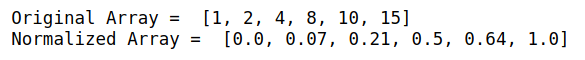## Normalization of 2D-Array

To normalize a 2D-Array or matrix we need NumPy library. For matrix, general normalization is using The Euclidean norm or Frobenius norm.

The formula for Simple normalization is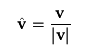Here, v is the matrix and |v| is the determinant or also called The Euclidean norm. v-cap is the normalized matrix.

Below are some examples to implement the above:

Example 1:

## Python3

 `# import module``import` `numpy as np` `# explicit function to normalize array``def` `normalize_2d(matrix):``    ``norm ``=` `np.linalg.norm(matrix)``    ``matrix ``=` `matrix``/``norm  ``# normalized matrix``    ``return` `matrix` `# gives and array starting from -2``# and ending at 13``array ``=` `np.arange(``16``) ``-` `2` `# converts 1d array to a matrix``matrix ``=` `array.reshape(``4``, ``4``)``print``(``"Simple Matrix \n"``, matrix)``normalized_matrix ``=` `normalize_2d(matrix)``print``(``"\nSimple Matrix \n"``, normalized_matrix)`

Output: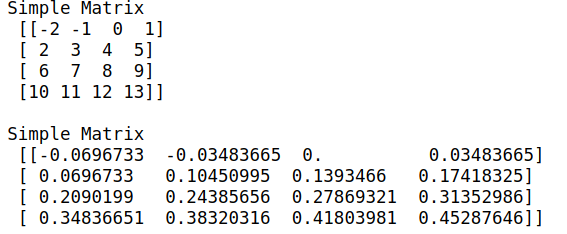Example 2:

We can also use other norms like 1-norm or 2-norm

## Python3

 `# import module``import` `numpy as np` `def` `normalize_2d(matrix):``    ``# Only this is changed to use 2-norm put 2 instead of 1``    ``norm ``=` `np.linalg.norm(matrix, ``1``)``    ``# normalized matrix``    ``matrix ``=` `matrix``/``norm ``    ``return` `matrix` `# gives and array starting from -2 and ending at 13``array ``=` `np.arange(``16``) ``-` `2` `# converts 1d array to a matrix``matrix ``=` `array.reshape(``4``, ``4``) ``print``(``"Simple Matrix \n"``, matrix)``normalized_matrix ``=` `normalize_2d(matrix)``print``(``"\nSimple Matrix \n"``, normalized_matrix)`

Output: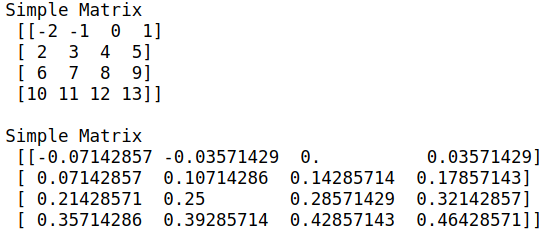In this way, we can perform normalization with NumPy in python.

My Personal Notes arrow_drop_up